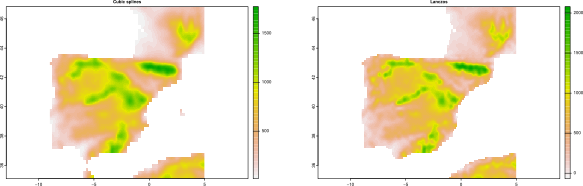### Introduction to R Spatial

3/24/22###### Víctor Granda-García
Ecosystem Modelling Facility - CREAF###### Roberto Molowny-Horas
Ecosystem Modelling Facility - CREAF

#### Course description

The aim of this course is to become familiar working with spatial data in R. In the first half of the course we will show how we can load and inspect vector and raster data from within your R session. This also includes basic vector operations, like e.g. spatial joins and filters, raster algebra (merge, extract…) as well as some basic visualization techniques. In the second half of the course, we will illustrate the use of spatial data in statistical analysis like e.g. raster interpolation, point pattern analysis and krigging.

#### Last course event

Last course event was hold at CREAF between 2022-03-14 and 2022-03-18

#### Materials:

01_introduction_to_vector_data.pdf

02_introduction_to_raster_data.pdf

03_introduction_to_spatial_statistics_toc.pdf

04_introduction_to_spatial_statistics_gbif_data.pdf

05_introduction_to_spatial_statistics_worldclim_data.pdf

06_spatial_homogeneity_of_point_patterns.pdf

07_spatial_autocorrelation.pdf

08_spatial_interpolation.pdf

09_spatial_correlation_structure_in_linear_regression.pdf

10_geographically_weighted_regression.pdf